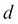# Turning a linear regression model into a curve – polynomial regression

In the previous sections, we assumed a linear relationship between explanatory and response variables. One way to account for the violation of linearity assumption is to use a polynomial regression model by adding polynomial terms:Here,denotes the degree of the polynomial. Although we can use polynomial regression to model a nonlinear relationship, it is still considered a multiple linear regression model because of the linear regression coefficients .

We will now discuss how ...

Get Python Machine Learning now with the O’Reilly learning platform.

O’Reilly members experience books, live events, courses curated by job role, and more from O’Reilly and nearly 200 top publishers.